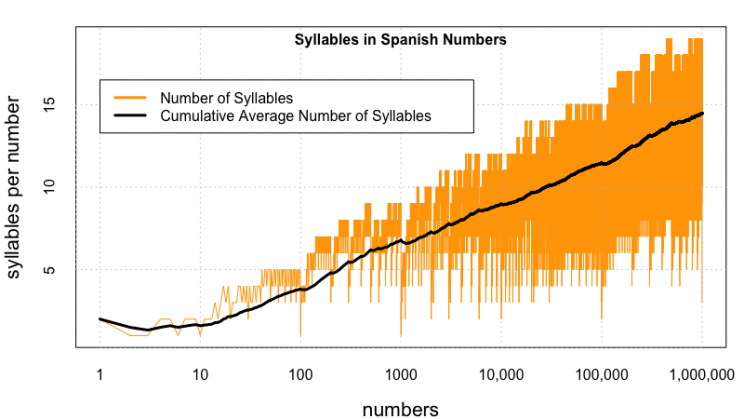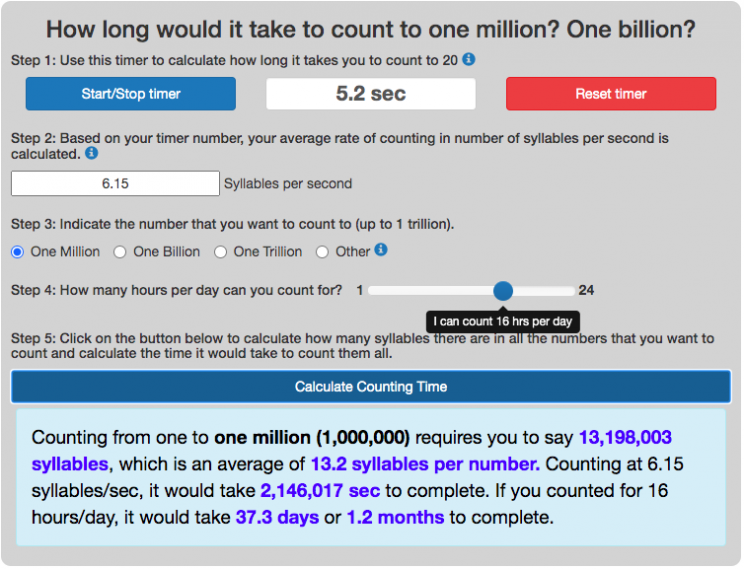# Posts for Tag: large numbers

### Counting to One Million, One Billion or One Trillion in Spanish

Posted In: Counting | ProgrammingThere was lots of interest in the calculator to estimate counting time (in English) to one million, one billion and up to one trillion.  I decided to do the same for other popular languages (Spanish).  Here is the calculator that will calculate how long it takes to count to one million (or larger numbers) in Spanish.  If you’d like to see this in Spanish click here.
(more…)

### How long does it take to count to 1 million? 1 billion? 1 trillion?

Posted In: Math | ProgrammingMy son likes large numbers (like septillion, googol and googolplex) and once asked me how long it would take to count to septillion (which is 1 followed by 24 zeros).  I told him it would take longer than the age of the universe to do that, so he started working his way down.  He asked me about counting to one million.  I did a little math (assuming one number per second) and got about 11-12 days . . . but then thought, the large numbers (like 658,243) take more than a second to say.

Looked on the web a little to see if anyone else had done a more sophisticated calculation.  Lots of calculations were like my own (assuming one number per second).  Others acknowledge that it would take longer for large numbers and made assumptions about what that would be.  But nothing definitive, so I thought I’d make one.  This counting calculator is based on the number of syllables in every number and counts all the syllables you’d have to pronounce in order to count from one to one million (or other numbers).